Density can sometimes be a tricky concept for students to understand. You can't easily estimate an object's density by simply looking at it. You have to think about the relationship between two of its properties: mass and volume.

In these activities, students will learn about mass, volume and density and their relationship to each other by conducting investigations into buoyancy: floating (and sinking) objects used in their everyday lives.

### Objectives

• Demonstrate how the distribution of molecules in a substance determines its density.

• Investigate the relative densities of liquids and the relative densities of solids.

• Predict, test and explain relative density by investigating the interactions of liquids and solids.

• Demonstrate understanding of the relationship between density and buoyancy by building a boat.

### Materials

• see individual activities for materials.

### Background

Density, Mass & Volume

Simply put, density is how tightly “stuff” is packed into a defined space.

For example, a suitcase jam-packed with clothes and souvenirs has a high density, while the same suitcase containing two pairs of underwear has low density. Size-wise, both suitcases look the same, but their density depends on the relationship between their mass and volume.
Mass is the amount of matter in an object.
Volume is the amount of space that an object takes up in three dimensions.

Density is calculated using the following equation: Density = mass/volume or D = m/v.

Let’s compare three familiar substances to explore the concept of density. If we take the same volume (one cubic centimetre) of foam, wood and concrete, we can see that each has a different mass.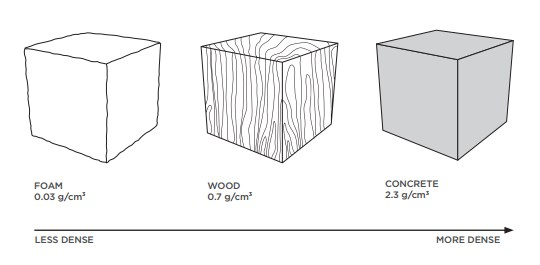Less Dense, More Dense

If something is heavy for its size, it has a high density. If an object is light for its size it has a low density.

A pebble is heavy for its size, compared to a piece of popcorn which is light for it’s size.  Imagine a big bowl of popcorn, compared to a big bowl of pebbles, which would feel heavier?

It is easy to estimate relative densities if you keep either the volume or the mass of two objects the same.

If you filled one bag with a kg of feathers and another with a kg of lead you would see that the feathers take up much more room, even though both bags have the same mass. This because feathers are less dense, they have less mass per volume. If you made a copper cube and an aluminum cube of the same volume and placed one in each hand, you would be able to feel that the copper cube would be heavier. Copper has more mass per volume than aluminum.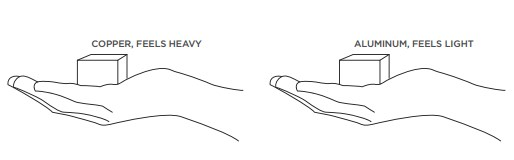How can one substance have more mass per volume than another? There are a few possibilities:

1. Atoms of one substance might be a similar size yet have more mass than the atoms of another substance.
2. Atoms of one substance might be a similar mass but be smaller, so more of them fit within the same volume.
3. Atoms of one substance might be arranged in a way that allows more of them to fit in the same volume.

Any one or a combination of these explanations could be the reason why one substance has a higher density than another. In the case of copper and aluminum, their atoms are arranged similarly, but copper atoms are smaller and have more mass than aluminum atoms, giving it a higher density.Density, Sinking and Floating
Why do some things float, while others sink? You might expect heavier objects to sink and lighter ones to float, but sometimes the opposite is true. The relative densities of an object and the liquid it is placed in determine whether that object will sink or float. An object that has a higher density than the liquid it’s in will sink. An object that has a lower density than the liquid it’s in will float.

You can really see relative densities at work when you look at a heavy object floating and a lighter one sinking. For example, imagine putting a small piece of clay and a large, heavy wax candle in a tub of water. Even though it’s lighter, the piece of clay has a higher density than water and therefore sinks. Even though it’s heavier, wax has a lower density than water, so the big candle floats.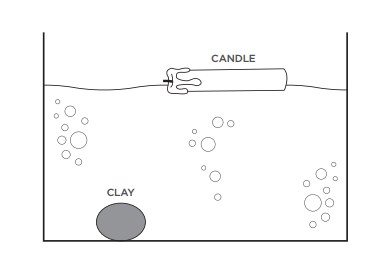Sinking and floating applies to liquids too. For example, if you add vegetable oil to water, the oil floats on top of the water because the oil has a lower density than the water.

Buoyancy and Archimedes’ Principle
The ancient Greek philosopher Archimedes found that when an object is submerged in water, it pushes aside (or displaces) an amount of water with the same mass as the object.

The water pushes upward against the object with a force (buoyancy) equal to the weight of water that is displaced.

Let’s explore Archimedes’ principle by dropping a bowling ball into a tub of water. When the ball is submerged in the water, it displaces its volume in water. According to Archimedes’ principle, the water can “push back” with a force equal to the weight of the water that has been displaced.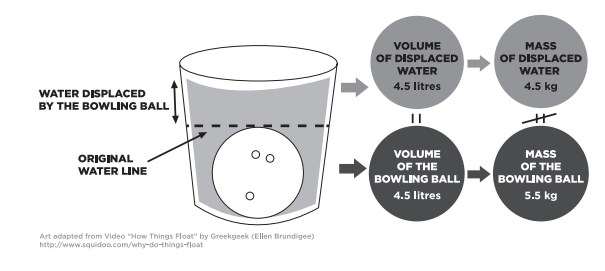A litre of water has a density of 1 kilogram per litre (1 kg/L), so a bowling ball’s worth of water (4.5 L) can push back on the bowling ball with a force equal to 45 newtons (N). That’s the weight of a 4.5 kg mass. However, the weight of the ball is more like 55 N. That’s more than the buoyant force of the water it displaced, so it sinks.

A beach ball may have the same volume as a bowling ball, but it has a much smaller mass. When you a beach ball in a tub of water, it displaces the mass of water equal to its own mass—about 0.01 kg. If you were to try to push the beach ball down and displace more water, the water would push back with a force greater than the weight of the beach ball. The push of the water keeps the beach ball afloat.

Buoyancy is the upward force we need from the water to stay afloat. Buoyant forces are why we feel so much lighter when we are in a swimming pool. Our bodies are mostly water, so our density is fairly close to that of water. Because of this, an average person needs only a little bit extra buoyancy to float. A life jacket provides this extra lift.

Changing Density
You can change the density of a substance by heating it, cooling it, or by adding something to it. If an object sinks in water, it’s because the object has a higher density than the water. There are two possible ways to make that object float, however:

1. Increase the density of the water so that the water becomes denser than the object. For example, an egg will usually sink in a glass of water, because it is denser than water. Adding salt to the water increases the density of the water, allowing the egg to float. This experiment also works with people, but you need a lot of salt (try the ocean, or even better, the Dead Sea !)2. Increase the volume of the object so that the object becomes less dense than the water. A great example of this is ice floating in water. Ice is formed by freezing water. When it freezes, it increases in volume as the water molecules move farther apart to accommodate the lattice structure of ice. Because the ice is now less dense than water, it floats. This phenomenon also explains why ships float even though they are made of steel. A ship is built in such a way that it encloses large amounts of open space. The ship still displaces its weight in water, but because of the way the ship is constructed, it takes up more space than the volume of the water it displaces, so it floats.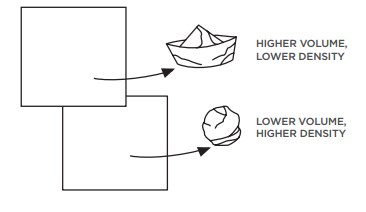### Vocabulary

Archimedes: Greek mathematician, physicist, engineer, inventor and astronomer (c. 287 BC–c. 212 BC).
Archimedes’ principle: Any object wholly or partially immersed in a fluid is buoyed by a force equal to the weight of the fluid that is displaced by the object. In other words, the buoyancy is equal to the weight of the displaced fluid.
buoyancy: The upward force that a fluid exerts on an object less dense than itself; the ability to float.
density: How closely packed together the molecules of an object or substance are.
displace: To push out of the way. For example, when an object goes into water, it displaces the water.
immiscible: Unable to be mixed together, like oil and water.
ironwood: The name for a large number of woods that have a reputation for hardness and high density.
mass: The amount of matter in a given space.
matter: The substance that makes up all physical things.
pumice stone: Lava froth known for its small mass and low density, despite looking like a rock.
weight: A measure of the force of gravity on an object.
volume: The amount of space a substance or object takes up.

### Other Resources

BrainPOP | Science | Matter & Chemistry | Measuring Matter

EDinformatics | Mass, Volume, Density

WatchKnowLearn.org | Buoyancy and Density

ProTeacher Collection | Density